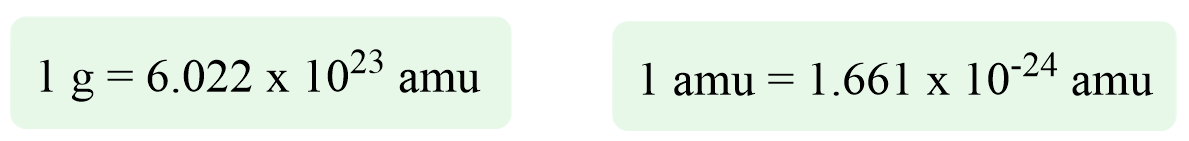# Atomic Mass

Because atoms and molecules are extremely small and we cannot weigh and work with them directly, there are a few ways you will see expressing their masses. This may be confusing at first but doing some practice and pointing out to what is going to be used the most, should help clarify these terms.

Let’s start with the ones you are going to see most often. Atoms have their own unit for mass, which is called atomic mass unit (amu). One amu is defined as the one-twelfth of the mass of one 12C atom. The masses of other atoms are reported relative to this standard. For example, from the periodic table, we can find that the atomic mass of O is 15.999 amu, for Cu, it is 63.546 amu, for S it is 32.06 amu, and etc.

The correlation between atomic mass units and grams is determined to be:The good news is that you will most likely not need to remember and work with these extremely large and small numbers in yours in your class.

For now, keep in mind the atomic mass units which you can find, for each element, in the periodic table.

# Average Atomic Mass

You may be wondering why the masses are not whole numbers, and this is because the periodic table shows the average mass of all the isotopes for the given element. Remember, isotopes have a different number of neutrons and because the atomic mass is the sum of the masses of all the protons, neutrons, and electrons, their atomic masses are also different.

The average atomic mass is calculated considering the mass and the abundance percentage of each isotope. To calculate the average atomic mass, we multiply the mass of each isotope with its percent fraction and get the sum of this product for all the isotopes.

For example, copper has two naturally occurring isotopes: 69.17% Cu-63 with a mass of 62.9291 amu and 30.83% Cu-65 with a mass of 64.9278 amu.

The average mass of Cu is:

Average atomic mass (Cu) = 0.6917 x 62.9296 amu + 0.3083 x 64.9278 amu = 63.55 amu

So, keep in mind, whenever, we say, for example, the atomic mass of carbon is 12.01 amu, we are talking about its average atomic mass, and this is what you find in the periodic table.

# Molecular Mass

Molecular mass is the mass of a given molecule and is calculated by adding the atomic masses of the elements it consists of.

For example, the molecular mass of water is:

Molecular mas (H2O) = 2 x 1.0 amu + 16.0 amu = 18.0 amu

The molecular mass of Ba(NO3)2 is:

137.33 amu + 2 x 14.007 amu + 3 x 2 x 15.999 amu = 261.338 amu

Now, in the laboratory, we most often work on the grams or milligrams scale, and expressing the mass of a few-grams sample is not convenient using atomic mass units, there is what is called the molar mass. This is the mass of a one-mole sample, and we will talk about it in detail in the next article.

Check Also